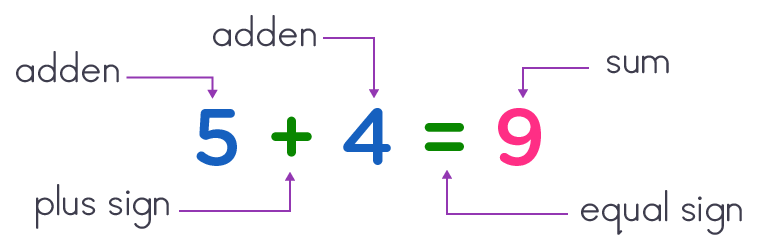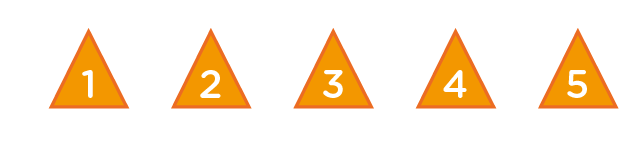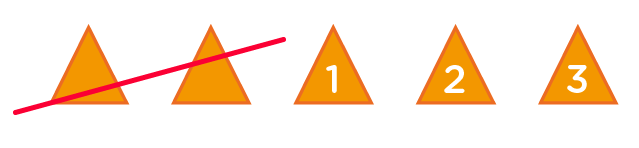# Addition up to 5 worksheets - Free Addition Worksheets for Kinders

## Understand the basic techniques of addition

Addition concept is one of the main pillars of math. 1 to 5 are very basic math numbers, thus addition up to 5 worksheet is a fun and interactive math practice that gives kids an excellent basic math foundation.

Having had a solid grasp of what a number is and what it represents, parents should not hesitate to obtain for their kids, our free addition worksheets for kinders. Our main objective in these worksheets is for kids to perfectly understand the basic techniques of addition.

###1 KINDERGARTEN MATH PRINTABLES

The most enjoyable part of this basic addition by kinders is seen when they realize that they can actually count and come out with the correct answer.

Most importantly, when they are able to really see the relationship between numbers and how their quantities relate to one another.

As kids begin to engage in our addition up to 5 worksheets, we want to make them see addition sentences in four parts; i.e. the two values called addends, the plus sign, the equal sign and the sum, called the answer.

An example of addition sentence can the illustrated thus: →### Avoid math anxiety and Gain early math confidence - addition up to 5

It is of vital importance that we continue to enhance the idea of number sense in kinders. With an innate idea already of what numbers mean, kids can fill anxious. As they engage in our inspirational free addition worksheets for kinders, they will avoid math anxiety and gain early math confidence.

One way of gaining kids love and confidence in our addition up to 5 activities is by formulating simple exercises with models, so that they can easily understand the basic technique of addition.

One of such basic techniques is representing the number of addends with visual objects. This will greatly help kids to easily add up by counting all the objects together, then obtain the correct answer.

Also, another technique used in our addition up to 5 worksheets is by formulating an addition sentence with one missing addend.

### Example: in each case, fill in the missing numbers

Addition - extremely simple – all about counting, counting and counting numbers or objects. Hey, let’s take a look at this one.

in each case, fill in the missing numbers.Ok. Let’s first of all take a look at the sentence. Hmmm! Only the sum and one addend is available in the addition sentence. One addend is missing, so the following guides will help us fill in that missing number.

1. Firstly, we move straight to the sum, and represent its number with objects.

The sum number is 5, so let’s go    →1. Secondly, given that the number of the one present addend is 2, we move over to our 5 objects, then count and cross out 2 objects from there. Let’s go  →1. Good! We can now count the remaining objects that have not been crossed out. The uncrossed objects will become our missing addend. Let’s count →1. Finally, having in mind that our last counted number names the number of all the objects, then there are 3 objects. Therefore, our missing addend or number is 3
2. We can now fill in our missing number: 3
3. So we have, 3 + 2 = 5

Wow! This is so wonderful. It’s very easy.

Have fun and enjoy math as you count and solve the rest of our simple exercises.

•